×

Search anything:

# SpineNet

#### Machine Learning (ML) Deep LearningGet this book -> Problems on Array: For Interviews and Competitive Programming

In this article, we introduce SpineNet as an image recognition deep learning Convolutional Neural Network (CNN). As a background, it is recommended that if you are not familiar with CNN, you should read this article first.

CNN is a relatively advanced machine learning (ML) topic but this blog post contains a bit-size introduction to different basic key operations of CNN and Artificial Neural Network (ANN) that is used for deep learning in the field of image recognition.

SpineNet proposes an alternative to ResNet50, a variant of the ResNet model which uses 50 layers of deep convolutional network (hence "50" in its name). It intends to disrupt the CNN architecture from a high level which has not changed over the years. In this essay, we will go through the core idea, model architecture, applications and accuracy of the SpineNet model based on this paper.

## 💡 Introduction and core idea

How does the SpineNet model differ from our typical ResNet50 architecture? Recall ResNet50 is simply 50 layers of Deep Convolutional network stacked together in a meticulously designed fashion.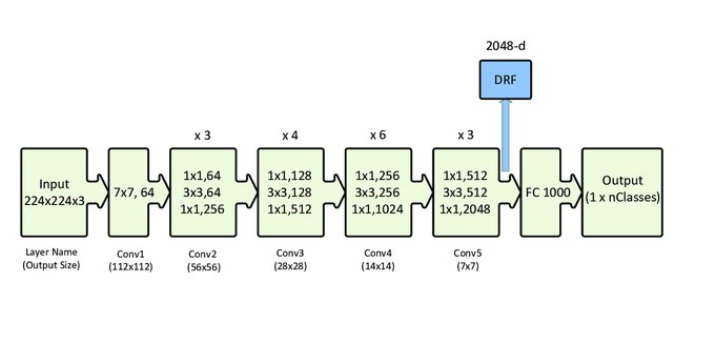Do notice the dimension of each layer, we started with 224 x 224 x 3 (3 stands of RBG colors), after the first convolution, it was reduced to 112x112, but with 64 layers. It further reduces it size of the image but increases its depth. A brief overview of the CNN architecture (similar to Resnet50) is shown below: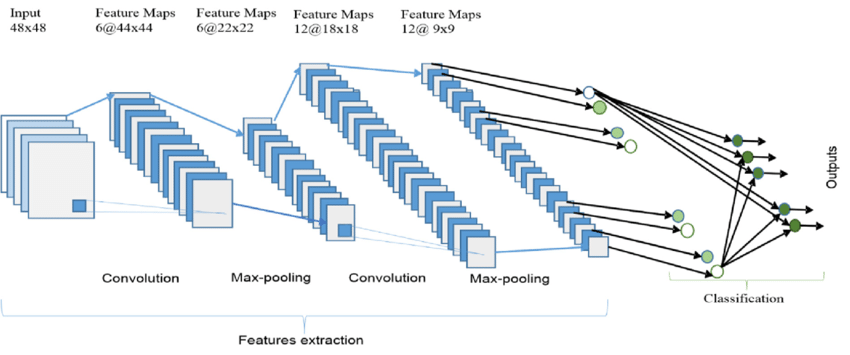The idea here is every time it goes through the convolution, features are detected while preserving the spacial features of the images. Below is an example of such idea on feature extraction .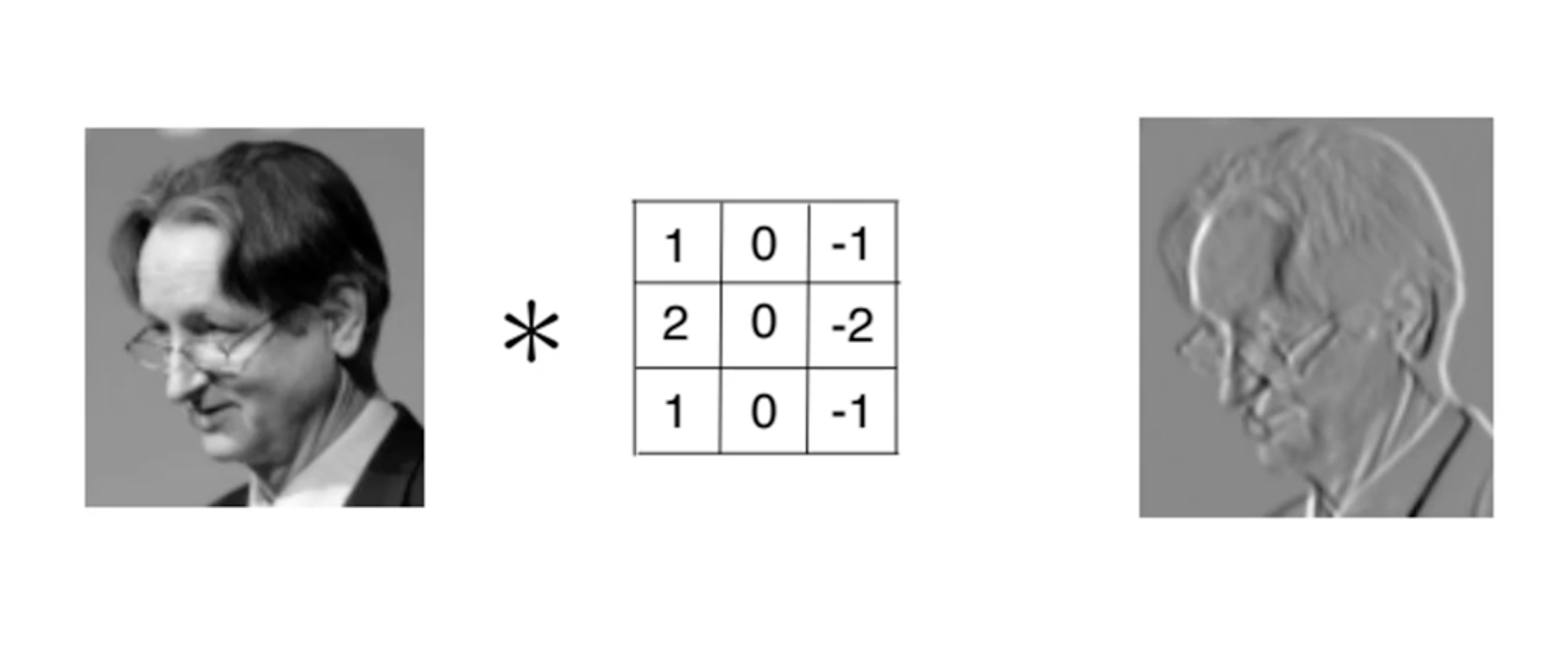At the end of the CNN, the small-sized images would all contains feature extraction from the original picture and they would be effective in contributing towards recognizing objects in images, be it image classification (cat or dog) or more advanced recognition (bounding box detection). This traditional top-down approach has been around for decades and SpineNet studies the approach differently . Instead of linking the convolutional network from scaled-decrease network (large image size but small number of features to small image size but large numbers of features), it proposes a scaled-permuted network (means permute and rearrange the convolutional blocks and allow up to 2 connections in to and out of each block from other blocks not immediately adjcent to it)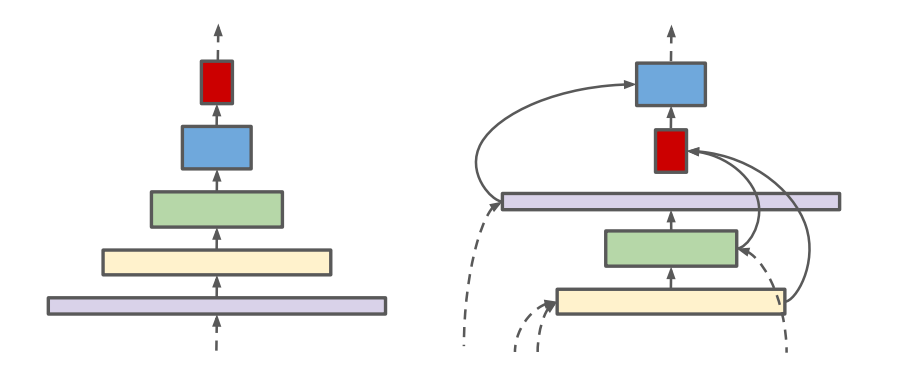We shall dive deeper into model architecture in the following section.

## 🗿 Model architecture

The model of SpineNet is under development. One may wonder how the actual permutation of the CNN layers is arranged since it the connections are all "messed up".

The rule of thumb here is the layers ddonot have to be linearly and orderly connected and arranged. They can exist in any order as long as the matrix multiplication allows, and each layer can have up to two connections to and from itself. The following is some proposal of the SpineNet architecture.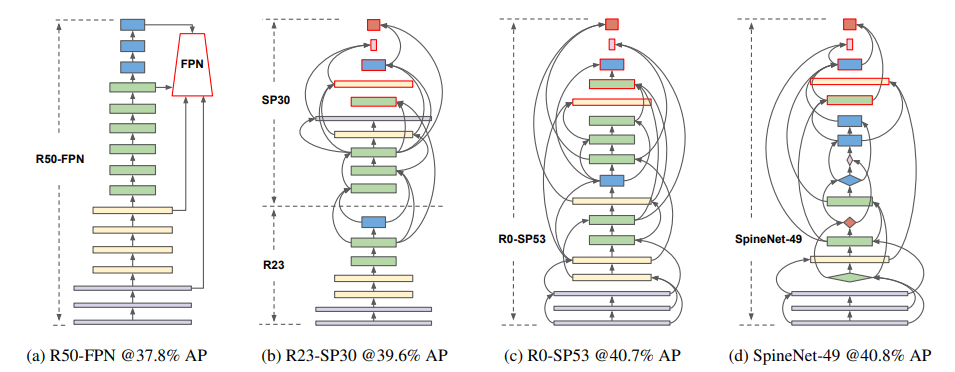The above figure  demonstrates a high-level idea of CNN connection from ResNet50 slowly to scaled-permuted networks. On the CNN at (a), it represents a normal ResNet50 CNN architecture. (b) is a partially scale-permuted network with R23 is traditional CNN and (SP30) as permutated. The rest of the model (c) and (d) is connected in such a way that they do not follow any traditional connection like ResNet50. While containing considerable cross-connections between all the layers, the authors from  found that model (c) and (d) performs the best after permutation from ResNet50. Model (c) specifically preserves the number of features and the type of blocks in each layer, while model (d) the model was tuned such that the number of features and types of blocks is optimized for minimal computation required. Notice the different shapes in the blocks for model (c). The diamond-shaped blocks are called bottleneck blocks while the rectangular block is residual blocks (residual is what normally used in ResNet50, read more about it here). A Bottleneck Residual Block is simply a variant of the residual block that utilizes 1x1 convolutions layers to create a bottleneck. The use of a bottleneck reduces the number of parameters and matrix multiplications. The idea is to make residual blocks as thin as possible to increase the depth and have fewer parameters. That saves some computation when the model chooses to use bottleneck block versus regular residual block. We would discuss the accuracy and computation runtime in the later section.

One may be tempted to ask, how does one decide how to connect these layers? The paper  suggests using neural architecture search with Reinforcement Learning (RL). You can read more about RL here.Basicallyy it means the layer organization and connection is randomized at the first. An agent will randomly build the model and define the state, then the model will be run and the accuracy would be the reward signal feeding back to the agent after every training, and it can do adjustments on its layer ordering and connection accordingly. However, the RL technique used in training SpineNet is quite complicated therefore this article will not dive deeply into how to design the RL to achieve optimal results for SpineNet. The author of the paper  trains the SpineNet by RL to improve the model accuracy.

However, one might doubt how the SpineNet is able to connect the two layers together with different dimensions. It is one of the challenges that the author face when designing the layers.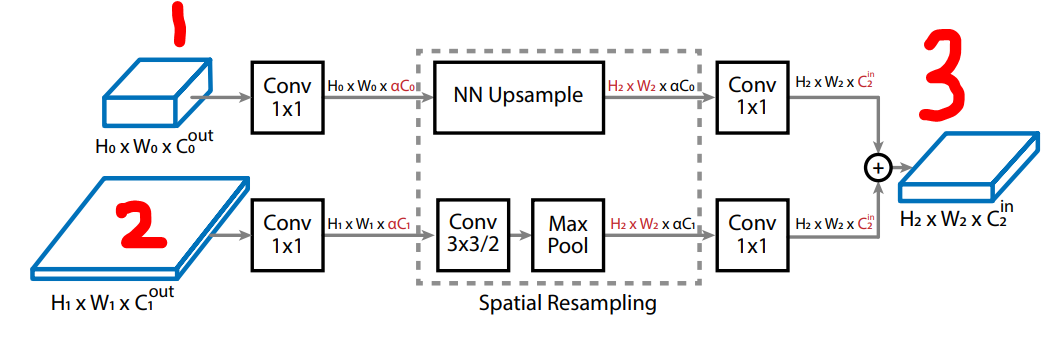Notice that in the above figure, layer 3 is connected to layer 1 and 2 since every layer can receive input from up to two layers. The number of features, C, is different for both 1, 2, and 3. The author connects the three items by first going through 1x1 convolution which was introduced by the original ResNet paper. In a nutshell, the 1x1 convolution network is a learned transformation from a number of input channels to a number of output channels without doing any actual convolutional operation. It is simply a linear operation that up-scales or down-scales the original input to desired output dimension. Then the two inputs are added back together while maintaining its dimension .

To summarize the SpineNet vs Original ResNet50, the SpineNet proposes the importance of:

• Scaled Permutation of blocks and layers
• Cross-scale connection between blocks and layers

We shall talk about the result of such an endeavor.

## 🎯 Accuracy of SpineNet

There are a lot of models investigated in the paper but we will stick to these four models:Comparing to the original ResNet (a) which achieved 37.8% average precision (AP), we can see a progressive improvement on models across (b), (c) and (d) with 39.6%, 40.7% and 40.8% AP. Notice that the AP improvement of (c) and (d) is minimal, but the bottleneck block at (d) requires less computation (around 10% compared to model (a)) and thus model (d) is ideal for maximum precision while requiring minimum calculations.

## 🔨 Applications

There is never-ending applications on CNN architectures, and a more accurate model requires less computation never hurts. Their applications include:

• object detection
• image classification
• Facial recognition

With this article at OpenGenus, you must have the complete idea of SpineNet.